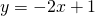# Solution assignment 05 Tangent line to graph

### Assignment 5

Calculate the equation of the line through the point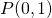parallel to the line: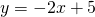### Solution

We can calculate the equation of this line with the formula: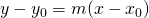with:The line has to be parallel to the line:which has a slope equal to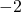. The required line must have the same slope (parallel) and thus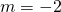.
The equation of the required line is: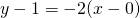or: